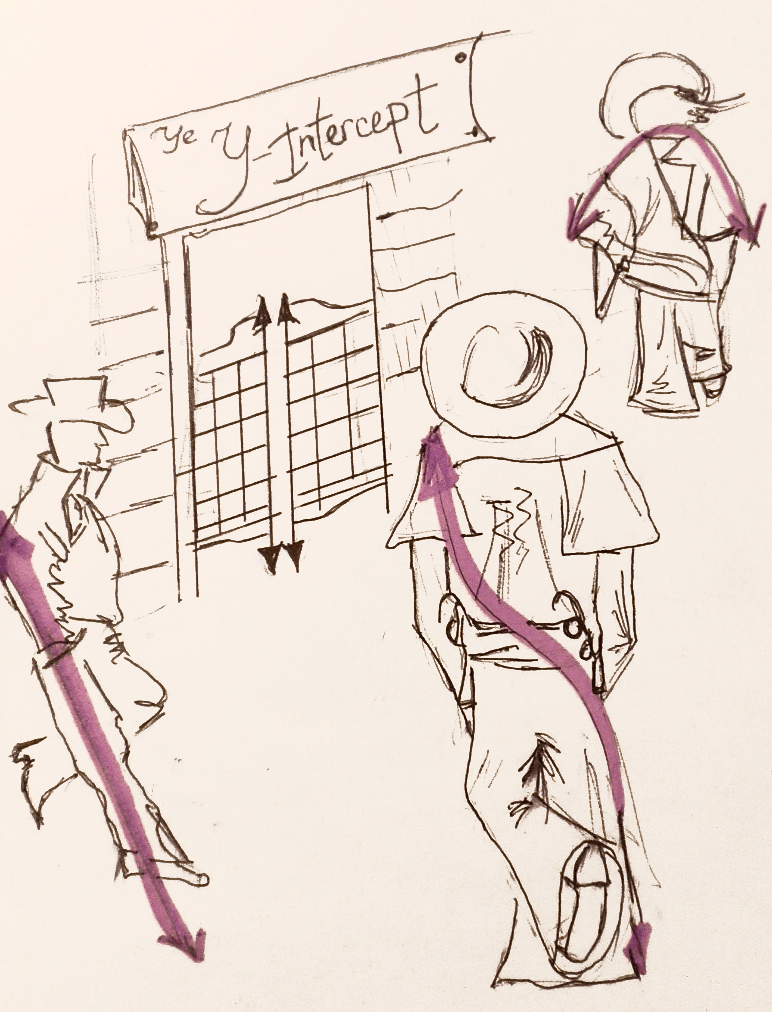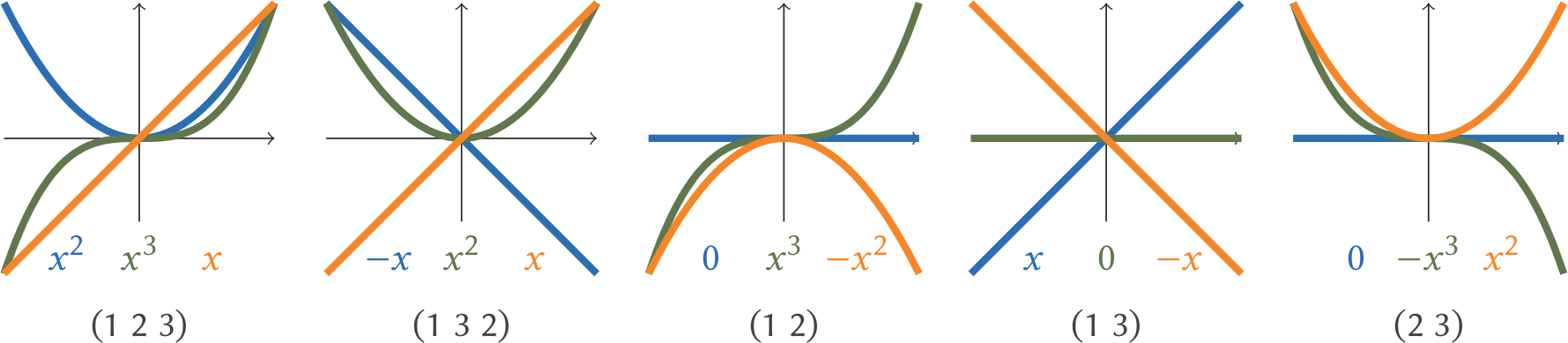# Polynomials’ order

Yuliya Nesterova orders some polynomials aroundThree polynomials walk into a bar. Well, a $y$-axis, actually. In all seriousness, suppose three distinct polynomials approach the axis $x=0$ from the left: one polynomial is bound to be on top, one on the bottom…Are they guaranteed to come out the other side in much the same way? And if not, how can we permute them? And if we may permute them, are all permutations possible? How many are possible?

Suddenly, the problem becomes far less a joke and far more a recently-closed problem. It was only in 2013 that Étienne Ghys demonstrated it in its generality: a permutation is realisable by polynomials if and only if it avoids being one of two types. Let’s make our way to the intersection, order up a drink, wiggle some polynomials, and explore the mystery!

## The origin

Formally, we wish to know if it is possible for three polynomials to cross the $y$-axis in one configuration and come out the other side with their order switched. By adjusting our polynomials up and down as necessary, we can restate the problem to be about polynomials crossing through the point $(0,0)$. Let us label the top polynomial to the left of the $y$-axis as ‘1’, the one below it as ‘2’, and the bottom one as ‘3’: in what order do ‘1’, ‘2’, and ‘3’ emerge on the right side of the axis? Using cycle notation, we can keep track of these wiggling polynomials.

Dipping into a bit of group theory, a permutation $\sigma \in S_n$ is code for a rearrangement of the numbers $1,2,3,…, n$. The symmetric group, $S_n$, is a much studied object: we can detect it when looking at the set of all possible permutations, or studying the symmetries of an $n$-simplex, and a whiff of it is present in many more maths problems.These polynomials give the permutation $(1\;3\;2)$

To every configuration of polynomials entering the origin from the left as $1, 2, 3$ and emerging in some new order $\sigma(1),\sigma(2), \sigma(3)$ so that the new position of 1 is $\sigma(1)$ etc, we may attach a permuation $( 1\; \sigma(1)\; \sigma(\sigma(1)) )$. For example, for the polynomials in the picture on the right, $1,2,3$ left of the origin becomes $2,3,1$ on the right as $x^3 – 3x$ dips from top place to come out on the bottom on the right, so the permutation here is $$(1 \ \sigma(1) \ \sigma(\sigma(1))=(1 \ 3 \ \sigma(3)) = (1 \ 3\ 2).$$ Are all 6 permutations in the symmetric group $S_3$ of 3-object permutations achievable?

A short search can find all 6 such 3-configurations. In fact, we need look no further than cubics to observe all possibilities. Here they are in full:## Possibilities with four polynomialsIn 2009, Maxim Konsevitch had shown the following: the permutations $(1\;3\;4\;2)$ and $(1\;2\;4\;3)$ cannot be realised by polynomials. All other 22 permutations of $\left\{ 1,2,3,4 \right\}$ can be realised, yet these 2 mysterious configurations are not possible to achieve with polynomial functions. To prove the permittable permutations is to write down 22 cases: an engaging activity of which it would be criminal to deprive the reader. To prove the impossibility of our mysterious pair, we will need to pull the concept of a valuation from our algebraic minibar.

Suppose $f(x)=a_n x^n +…+a_1 x + a_0$ is a real polynomial: a polynomial with real numbers for coefficients. The valuation of $f$ at $x=0$, $v(f)$, is the smallest $i$ with $a_i\neq 0$. For example,

$$v(8x^{2} + 20x)=1$$
and
$$v(x^{20} + 600 x^{10} – x)= 1.$$

Close to the origin, the smallest terms serve the most decisive role. The valuation of a constant is defined to be $\infty$.

If you have yourself been gathering the 22 polynomial cases, you have perhaps noticed that it is useful to treat the $x$-axis itself as a polynomial, simplifying the problem. (To achieve this, we may pick a polynomial $f_k$ to straighten out and replace all polynomials $f_i$ under consideration by $f_i – f_k$. For the purposes of our proof, pick $f_k$ to be $f_4$, one that’s the bottom of the bunch to the left of the axis.)$(1\; 3\; 4 \; 2)$ gives us trouble

Imagine the four polynomials ambling from left to right in time, crossing over the origin and switching places in the process in accordance with the target permutation $(1\;3\;4\;2)$. Under our assumption, to the left of the origin and before the mixing, $f_4$ is at the bottom (our newly minted $x$-axis) and the other 3 polynomials are above. Since $f_4$ goes to second place under $(1\;3\;4\;2)$, we must have 2 polynomials which were previously above $f_4$ for $x<0$ but now are below for $x>0$. Making use of our assumption that $f_4$ is the $x$-axis, we look for 2 polynomials (our $f_1$ and $f_3$) which cross the $x$-axis at the origin, with $f_1$, now in 3rd place, on top of the $f_3$, now fourth.

Crucially, we may observe that close to the origin, the non-constant polynomial with $v(f)=n$ is approximated by $a_n x^n$ : all other terms go to zero faster than the valuation’s term. But what of our valuation? It is useful to us precisely for the fact that $f(x)$ crosses the $x$-axis at the origin if and only if $v(f)$ is odd. And by definition it is the odd functions which have rotational symmetry and switch sign either side of the origin. Thus, near the origin, if $n$ is odd and $a_n$ is positive,

$$f(x) \approx a_n x^n \text{ which is }\begin{cases} >0 & \text{for }x<0,\\ <0 & \text{for }x>0. \end{cases}$$

What of it? We now have established $v(f_3)$ and $v(f_1)$ to be odd and $v(f_2)$ to be even. The parity is different. Let’s look at the order in which these polynomials appear more closely.

To the left of the origin,

$$f_1(x)>f_2(x)>f_3 (x) >0$$

by our choice of indices; to the right, $f_2(x)>0>f_1(x)>f_3(x).$ And near the origin, in the good company of fractions, each function looks much like its valuation-exponent power of $x$. Inching closer to $(0,0)$ as necessary, we find $v(f_1)\geq v(f_2)\geq v(f_3)$. We had glimpsed earlier that $f_1(x)>f_3(x)$ to the right of the origin, so $v(f_3) \geq v(f_1)$, as the 3rd function decreases faster that the 1st.

There is only one way to interpret $v(f_1)\geq v(f_2)\geq v(f_3) \geq v(f_1)$: equality all round! Yet in the last paragraph, we noted the parity must be different. This leads us to a contradiction.

But must we repeat the same song and dance for the $(1\;2\;4\;3)$ configuration? No: it is enough to note that this is the opposite cycle. Take the picture we painted for $(1\;3\;4\;2)$, hold it up to the mirror, and see the impossibility of $(1\;2\;4\;3)$!

## Back for more (polynomials)

What wonders lie beyond groups of four polynomials crossing at the origin? In his 2013 paper Intersecting Curves, Étienne Ghys showed that every permutation in $S_n$ is realisable—provided that the permutation did not ask any subset of the four polynomials to alternate in the unachievable $(1\;3\;4\;2)$ and $(1\;2\;4\;3)$ configurations. Make sure those 2 permutations are not in your entourage and the polynomials can enter from the left and emerge to the right of (0,0) in any order they please! But if you pick four of them and try to force the bottom of the group to be second, and the third to be the bottom, you’ll have an impossibility on your hands. You’ll be the butt of the joke!

It is easy to show necessity: erase all other polynomials but any four and we see that the forbidden permutations are as forbidden as ever. Yet is tricky to show this as a sufficient condition.

For this proof, we need graph theory: we must walk out of our pub at the origin and into a garden of pruned trees. We must build a tree for every collection of polynomials, starting from a constant-term-is-zero-for-all root, to linear terms and beyond, assigning a level to each degree and a node to each set of polynomials with identical terms of that degree. The leaves of such a tree will stand for the highest degree terms which help set all polynomials in a group apart. But alas, we must prune these trees if we have any hope that a computer may count the great many of them! Finally, by induction, we can describe, from a tree of $n-1$ polynomials, how to introduce the final $f_n$ and how we both can and cannot tack on an extra leaf. No $(1\;3\;4\;2)$ and $(1\;2\;4\;3)$ overlap will form.

Our tale ends here for now.A master’s student of algebraic geometry at Queen’s University, Canada, Yuliya likes to celebrate the informal mathematics that happens in the cracks of academia. She volunteers at MathQuest camp, a mathematics camp for girls, creates Pi Day celebrations, and hosts Ottawa’s MathsJams.
@ottawamathsjam    mathquest.ca    + More articles by Yuliya